## Vba charts introduction, all the chart types in Excel

In Excel, a chart is a visual representation of data from a worksheet that gives a more detailed graph, rather than, simple text

A chart is sometimes called as a graph.

It is a powerful tool that enables the user to visually present the data with much more analytical details.

Charts are very often used with excel for data presentation.

For illustration purpose, let us have a sample data as give below: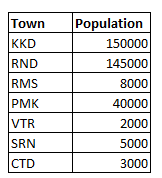Now, with reference to the above data, the various chart types are discussed as below

1.Line Chart

This chart is of linear type with x-axis and y-axis and the data are displayed as below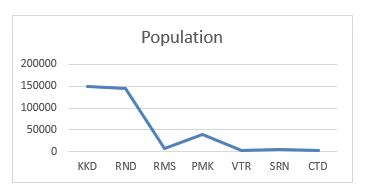2.Bar Chart

The same data are presented in a Bar chart as shown below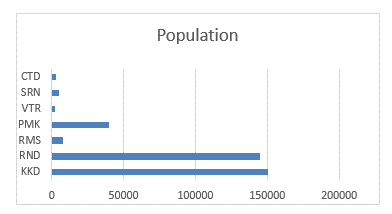3.Column Chart

This chart presents the data as separate columns for each data.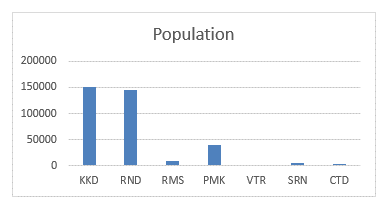4.Pie Chart

This chart is more convinient in presenting large data and is the most commonly used chart.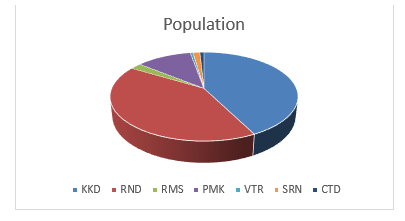5.Bubble Chart

The Bubble chart for the same data is as shown below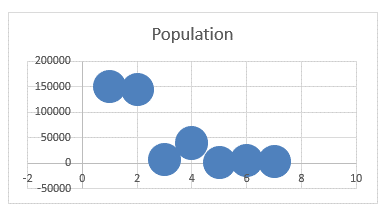It should be noted that, for every chart, there are multiple types, which can be chosen according to the need.